Fluid Mechanics
Volume 2, Issue 1, September 2016, Pages: 8-12

Thermal Instability in a Horizontal Layer of Ferrofluid Confined Within Hele-Shaw Cell

Ankuj Bala1, Ramesh Chand2, *

1Department of Mathematics, Dravidian University Srinivasavanam Kuppam, Chittoor, Andhra Pradesh, India

2Department of Mathematics, Government Arya Degree College Nurpur, Himachal Pradesh, India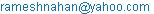(R. Chand)

*Corresponding author

Ankuj Bala, Ramesh Chand. Thermal Instability in a Horizontal Layer of Ferrofluid Confined Within Hele-Shaw Cell.Fluid Mechanics. Vol. 2, No. 1, 2016, pp. 8-12. doi: 10.11648/j.fm.20160201.12

Received: September 26, 2016; Accepted: October 19, 2016; Published: November 2, 2016

Abstract: Linear thermal instability analysis of a ferrofluid layer confined between in Hele-Shaw cell is investigated. The stability theory is based upon perturbation method and normal mode technique and the resulting equations are solved by using Galerkin weighted residuals method to find expressions for Rayleigh number and critical Rayleigh number. ‘Principle of Exchange of Stabilities’ hold and the oscillatory modes are not allowed in the problem. It is found that Hele-Shaw number delays the onset of convection while magnetization parameter and buoyancy magnetization parameter hasten the onset of convection.

Keywords: Ferrofluid, Perturbation Method, Galerkin Method, Hele-Shaw Number, Magnetization Parameter

Contents

1. Introduction

Ferrodynamics deals with the interaction of the magnetic fields on conducting, as well as non-conducting ferromagnetic fluids. Magnetic fluids also called ‘ferromagnetic fluids’ are electrically non-conducting colloidal suspensions of solid ferromagnetic magnetite particles in a non-electrically conducting carrier fluid like water, kerosene, hydrocarbon etc. A typical ferromagnetic fluid contains 1023 particles per cubic meter. These fluids are not found in nature but are artificially synthesized. The investigations on ferrofluids attracted researchers because of its applications in area such as instrumentation, lubrication, vacuum technology, metals recovery, bio- medical applications, acoustics etc. These fluids are widely used in sealing of hard disc drives rotating X-ray tubes under engineering applications. One of the major applications of ferrofluid is its use in medical fields such as the transport of drugs to an injured site and the removal of tumors from the body. Convection of ferromagnetic is gaining much importance due to their astounding physical properties and one such property is viscosity of ferrofluid. A detailed introduction to this subject has been given in the celebrated monograph by Rosensweig . This monograph reviews several applications of heat transfer through ferrofluids. Since magnetization depends on the magnetic field, temperature and density, any variation of the above causes a change in the body force of the fluid and gives rise ferro convection which is similar to Bénard convection given by Chandrasekhar . Finlayson  has studied the convective instability of ferromagnetic fluids, whereas thermo convective stability of ferrofluids without considering buoyancy effects has been investigated by Lalas and Carmi . Thermo convective stability of ferromagnetic fluids was continued by Blennerhassett et al. . Thermosolutal convection in ferromagnetic fluid was studied by Sunil et al. . Mahajan  studied the linear and nonlinear convective instability of a ferromagnetic fluid for a fluid layer heated from below under various assumptions. Recently Chand and Bala [8-9], Bala and Chand [10-12] studied the problem related to the thermal instability in a horizontal layer of ferrofluid by using Galerkin weighted residuals method. But in the present study, we studied the stability of ferrofluid layer confined within Hel-Shaw cell heated from below. Hele-Shaw cell is device, whose essential features are two parallel plates separated by an infinitesimally small gap containing a thin layer of fluid. Hele-Shaw flow finds its applications in various fields of sciences and engineering particular in matter physics and material science. The governing equations of the fluid flow in Hele-Shaw cell are similar to those governing the fluid flow in porous medium. Hele-Shaw  was first showed the analogy between flow in porous medium and Hele-Shaw cell by defining an equivalent permeability of b2/12 for the Hele-Shaw cell, where b is the width of fluid layer gap. Wooding  used free convection in Hele-Shaw cells to simulate thermal convection in porous medium.The objective of the present investigation is to study the onset of the convection ofa ferromagnetic fluid confined within a Hele-Shaw cell using Brinkman model.

2. Mathematical Formulation of the Problem

Consider a horizontal layer of an electrically non-conducting incompressible ferromagnetic fluid of height ‘d’, vertically confined between two parallel boundaries at z = 0 and z = d. Fluid layer shall be infinitely extended in the x-direction, but confined in the y-direction by vertical impermeable boundaries (side walls) at y = 0 and y = b (˂˂d). Fluid layer is heated from below in a porous medium of porosity is ε and medium permeability is k(= b2/12)in such a way that a uniform temperature gradient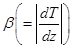is maintained, where T denote the temperature. The temperature T at z = 0 taken to be T0 and T1 at z = d, (T0 > T1). Let the system is acted upon by gravity force g(0,0,-g) and a uniform magnetic field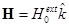also acts outside the fluidlayer.

2.1. Assumptions

The mathematical equations describing the physical model are based upon the following assumptions

ii.        Thermo physical properties of fluid expect for density in the buoyancy force (Boussinesq hypothesis) are constant for the purpose of characterization and estimates of the various effects on the order of magnitude.

iii.       Dilute mixture.

iv.      No chemical reactions take place in fluid layer.

v.        Negligible viscous dissipation.

vi.      Negligible radiative heat transfer.

vii.     Fluid is incompressibleand laminar flow.

2.2. Governing Equations

When the Hele-shaw cell gap width is not sufficiently small with regard to appearing wavelength of the instability, the correction to Darcy’s law is needed. Therefore on employing the Brinkman model, the governing equations under Hele-Shaw approximation for ferrofluid (Chandrasekhar , Resenweig  and Finlayson ) are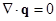(1)(2)

where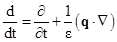stands for convection derivative, q(u, v, w) is the velocity vector, ρ0 is reference density, p is the hydrostatic pressure, k(= b2/12) is medium permeability of fluid, μ is viscosity of the fluid,μ0 is magnetic permeability, H magnetic field, M is magnetization.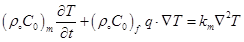(3)

where (ρ0C0)m is heat capacity offluid in porous medium, (ρ0C0)f is heat capacity offluid andkm is thermal conductivity.

Maxwell’s equations, in magnetostatic limit: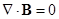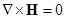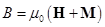(4)

The magnetization has the relationship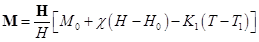(5)

Where B is magnetic induction, K1 is thermal conductivity,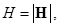and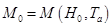.

The magnetic susceptibility and pyomagnetic coefficient are defined byand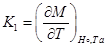respectively.

The density equation of state is taken as(6)

Where Ta is the average temperature given by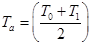.

The boundary conditions (Chandrasekhar ) are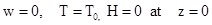and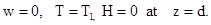(7)

2.3. Basic Solutions

The basic state is assumed to be a quiescent state and is given by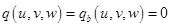,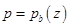,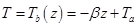,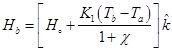,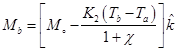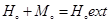(8)

2.4. The Perturbation Equations

We shall analyze the stability of the basic state by introducing the following perturbations: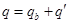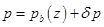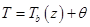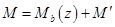(9)

where q′(u,v,w), δp, θ, H′(H'1,H'2,H'3) and M′(M'1,M'2,M'3)are perturbations in velocity, pressure, temperature, magnetic field and magnetization. These perturbations are assumed to be small. Then the linearized perturbation equations are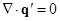(10)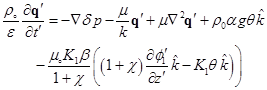(11)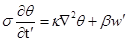(12)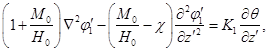(13)

where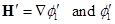is the perturbed magnetic potential,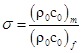and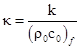is thermal diffusivity of the fluid.

The dimensionless boundary conditions are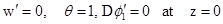and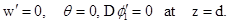(14)

Introducing non-dimensional variables as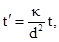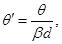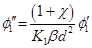,

Where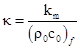is thermal diffusivity of the fluid.

Equations (10)-(14), in non-dimensional form can be written as(15)(16)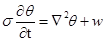(17)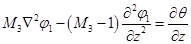(18)

[Dashes ( '' ) have beendroppedfor simplicity]

Here non-dimensional parameters are given as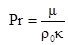is the Prandtl number;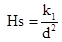is the Hele-Shaw number;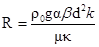is the Rayleigh number;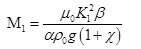measure the ratio of magnetic to gravitational forces;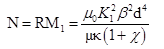is the magnetic thermal Rayleigh number;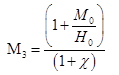measure the departure of linearity in the magnetic equation of state and values from onehigher values are possible for the usual equation of state.

Non- dimensional boundary conditions are given byand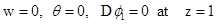.                (19)

On eliminating δp from equation (16), we have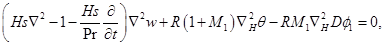(20)

where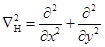is two-dimensional Laplacian operator on horizontal plane.

3. Normal Mode Analysis

Analyzing the disturbances into the normal modes and assuming that the perturbed quantities are of the form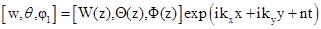(21)

Where kx and ky are wave numbers in x and y directions respectively, while n is the growth rate of disturbances.

Using equation (21), equations (20) and (17) - (18) become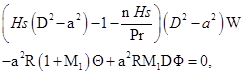(22)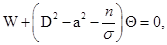(23)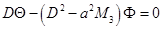(24)

where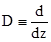and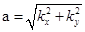is the dimensionless the resultant wave number.

The boundary conditions of the problem in view of normal mode analysis are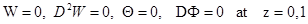(25)

4. Method of Solution

The Galerkin weighted residuals method is used to obtain an approximate solute on to the system of equations (22) – (24) with the corresponding boundary conditions (25). In this method, the test functions are the same as the base (trial) functions. Accordingly W, Θ and Φ are taken as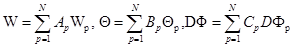(26)

where Ap, Bp and Cp are unknown coefficients, p =1, 2, 3,..., N and the base functions Wp, Θp and DΦp are assumed in the following form for free-free boundary conditions are:(27)

such that Wp, Θp and Φp satisfying the corresponding boundary conditions. Using expression for W, Θ and DΦ in equations (22) – (24)and multiplying first equation by Wp second equation by Θp and third by DΦp and integrating in the limits from zero to unity, we obtain a set of 3N linear homogeneous equations in 3N unknown Ap, Bp and Cp;p =1,2,3,..., N. For existing of non trivial solution, the vanishing of the determinant of coefficients produces the characteristics equation of the system in term of Rayleigh number R.

5. Linear Stability Analysis

We confined our analysis to the one term Galerkin approximation; for one term Galerkin approximation, we take N=1, the appropriate trial function are given as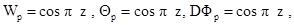(28)

which satisfies boundary conditions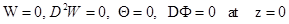and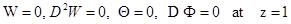.       (29)

Substituting solution (28) into equations (22)-(24), integrating each equation from z = 0 to z = 1, by parts and using boundary conditions (29), we obtain following matrix equation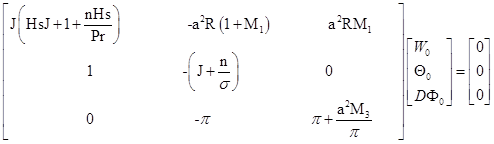where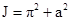.

The non-trivial solution of the above matrix requires that(30)

For neutral stability, the real part of n is zero. Hence on putting n = iω, (where ω is real and is dimensionless frequency) in equation (30), we have(31)

Where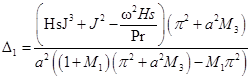(32)

and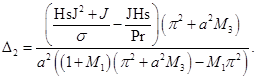(33)

Since Ris a physical quantity, so it must be real. Hence, it follow from the equation (31) that eitherω= 0 (exchange of stability, steady state) or Δ2 = 0 (ω ≠ 0 overstability or oscillatory onset).

But Δ2 ≠ 0, we must have ω = 0, which means that oscillatory modes are not allowed and the ‘Principle of Exchange of Stabilities’ is satisfied. This is the good agreement of the result as obtained by Finlayson .

Consider the case of stationary convection (n=ω = 0), from equation (30), we have(34)

In the absence of Hele-Shaw number i.e. Hs = 0, then equation (34) gives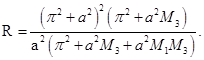This is the good agreement of the result as obtained by Finlayson .

In the absence of magnetic parameters (M1 = M3 = 0) and Hele-Shaw number (Hs = 0), the Rayleigh number R for steady onset is given by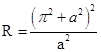(35)

Consequently critical Rayleigh number is given by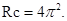This is good agreement of the classical result of Rayleigh-Bénard problem for Newtonian fluid.

6. Result and Discussions

In order to investigate the effects of magnetization parameter M3buoyancy magnetization M1 and Hs on the stationary convection, we examine the behavior of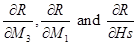analytically.

Equation (37), we have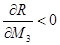, which mean magnetization parameter M3 has destabilizing effect on fluid layer. This is the good agreement of the result obtained by Bala and Chand [10-12].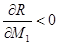, which imply buoyancy magnetization M1 destabilize the fluid layer. This is the good agreement of the result obtained by Bala and Chand [10-12].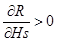which mean Hele-Shaw parameter Hs has stabilizing effect on fluid layer.

Thus Hele-Shaw parameter Hs has stabilizing effect while magnetization parameters M3 and buoyancy magnetization M1 have destabilizing effect on stationary convection of the fluid layer.

7. Conclusions

Thermal instability in a vertically oriented Hele-Shaw cell was investigated using linearly stability theory. Anexpression for Rayleigh number for the stationary convection is obtained by using Galerkin residual weighted method.

The main conclusions are as follows:

(i)       The magnetization parameters M3 and buoyancy magnetization M1have destabilizing effect while Hele-Shaw parameter Hs has stabilizing effect on stationary convection of the fluid layer.

(ii)      In the absence of magnetic parameters and Hele-Shaw parameter the obtained result is same as the result obtained by Chandrasekhar in the classical Bénard problem.

(iii)    The oscillatory modes are does not exist for the problem.

(iv)      The ‘Principle of Exchange of Stabilities’ is valid for the problem.

Acknowledgment

The authors would like to thanks the learned referees for their valuable comments and suggestions for the improvement of quality of the paper.

References

1. R. E. Rosensweig, Ferrohydrodynamics, Cambridge University Press, Cambridge 1985.
2. S. Chandrasekhar, Hydrodynamic and Hydromagnetic Stability, Dover, New York, 1961.
3. B. A.Finlayson, Convectiveinstabilityofferromagneticfluids, Journal of FluidMech., 1970, 40, 753-767.
4. D. P. Lalas and S. Carmi, Thermoconvective stability of Ferrofluid, Phys. of Fluids, 1971, 14, 436-437.
5. P. J. Blennerhassett, F. Lin and P. J. Stiles, Heat transfer through strongly magnetized ferrofluids, Proc. R. Soc. A, 1991, 433,165-177.
6. Sunil, P. K. Bharti and R. C. Sharma, Thermosolutal convection in ferromagnetic field, Arch. Mech., 2004, 56(2), 117-135.
7. A. Mahajan, Stability of ferrofluids: Linear and Nonlinear, Lambert Academic Publishing, Germany 2010.
8. R. Chand and A. Bala, On the onset of Rayleigh-Bénard convection in a layer of Ferrofluid, International Journal of Engineering Research and Applications, 2013, 3(4), 1019-1025.
9. R. Chand and A. Bala, Effect of rotation on the onset of Rayleigh-Bénard convection in a layer of Ferrofluid, International Journal of Modern Engineering Research, 2013, 3(4), 2042-2047.
10. A. Bala andR. Chand,Thermal instability in a horizontal layer of Ferrofluid in Brinkman porous medium,Journal of Scientific and Engineering Research, 2014, 1(2), 25-34.
11. A. Bala andR. Chand,Variable gravity effect on the thermal instability of Ferrofluid in a Brinkman porous medium, International Journal of Astronomy, Astrophysics and Space Science,2015, 2(5), 39-44.
12. A. Bala andR. Chand,Thermal instability in a horizontal layer of ferrofluid in anisotropic porous medium, Open Science Journal of Mathematics and Application, 2015,3(6), 176-180.
13. H. S. J. Hele-Shaw, Trans. Inst. Naval Archit, 40, 21.
14. R. A. Wooding,Instability of a viscous liquid of variable density in a vertical Hele-Shaw cell, Journal of Fluid Mech., 1961, 7,501-515.

 Contents 1. 2. 2.1. 2.2. 2.3. 2.4. 3. 4. 5. 6. 7.
Article ToolsAbstractPDF(211K)